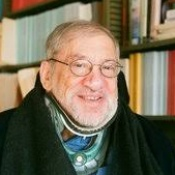# Michael Klass# Michael Klass

Professor Emeritus
Status
Current
Office / Location
319 Evans Hall
Phone
(510) 642-6550
Email
klass@stat.berkeley.edu
Research Expertise and Interests

statistics, mathematics, probability theory, combinatorics independent random variables, iterated logarithm, tail probabilities, functions of sums

My research interests are in probability theory. I have done research on sums of independent random variables, laws of the iterated logarithm, approximations of tail probabilities, operator limit theorems for sums of independent random vectors, expectations of functions of sums of fixed and random numbers of random variables, as well as arbitrary self-normalized sums.

statistics, mathematics, probability theory, combinatorics independent random variables, iterated logarithm, tail probabilities, functions of sums

Research Areas